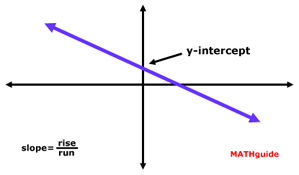Graphing Lines: Standard Form
View the Lesson | MATHguide homepage Updated April 7th, 2019

Status: Waiting for your answers.Graph the given line on your own paper then answer the questions that follow.

 Given: 5x + 3y = 60 Find: a) What is the location of the line's y-intercept?Write the location as an ordered pair (x,y).     (, ) b) What is the location of the line's x-intercept?Write the location as an ordered pair (x,y).     (, )